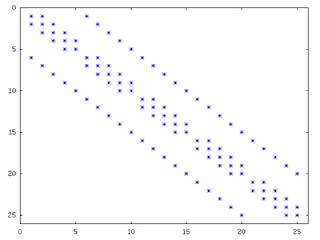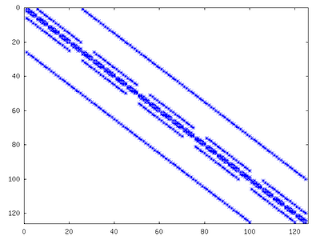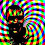## Sunday, January 11, 2009

### Kronecker Product of Sparse Matrices

Gilbert Strang discusses the use of a neat built-in function in Octave, kron(), that's pretty useful for doing multidimensional finite difference methods on PDEs:

kron() takes the Kroenecker product of two matrices. This is especially useful when constructing the large, sparse matrices needed for finite difference approximations.K is the tridiagonal matrix created for a central difference approximation to the second derivative:

n = 5;
K = spdiag(2*ones(n,1),0) + spdiag(-ones(n-1,1),-1) + spdiag(-ones(n-1,1),1);
spy(K)K is the differences for a 1D row of points, now we can create the 2-dimensional version for a plane of points with kron():

K2D = kron(speye(n),K) + kron(K,speye(n));
spy(K2D);The extension to 3 dimensions is exactly as before, now we have a matrix describing the differences between points on the same line in the first sub and superdiagonal, differences across lines in the next bands out from those, and differences across planes in the furthest out bands:

K3D = kron(speye(n),K2D) + kron(K2D,speye(n));
spy(K3D);

Pretty simple! Now you're ready to solve multidimensional partial differential equations.

1.There is an error in the code when going from the 2D system matrix to the 3D system matrix, should be:
K3D = kron(speye(n),kron(speye(n),K)) + kron(K,kron(spye(n),speye(n)) + kron(speye(n),kron(K,speye(n));

1.This isn't going from 2D to 3D, right? This looks like it goes straight from 1D to 3D. That is, I don't see anything that looks like K2D inside of K3D.

2.Yes; sorry for the sloppy wording.

I haven't touched this one in a while, happy to hear correctos or improvements.

3.Working on a 3D Laplacian constructor for the case where the image volume isn't isometric, i.e. volume.shape is (n1,n2,n3) instead of (n,n,n). I'm new to kron, so I may be making it more difficult than it needs to be. I'll post a link to the code here when I'm finished. Thanks for getting me this far!

4.I finished the function, but it turns out that I'm not allowed to post it publicly. Email me, and I'll send it to you, and you can feel free to post it wherever you like.

5.I would just post it to this thread; if your management isn't comfortable with you doing that it seems unlikely they'd appreciate me doing the same thing.

If you can't post code, this is a simple enough problem that narrative describing your solution would probably still be helpful to whoever comes across this next.

Thanks for commenting anyway. I'm glad you got to a solution, and that this post was some little help along the way.

2.Strang wrote far and away my favorite undergrad linear algebra book. His enthusiasm is infectious.

3.I have to agree; you can just sit down and read that one for fun. I'm really glad MIT put all these lectures of his up.